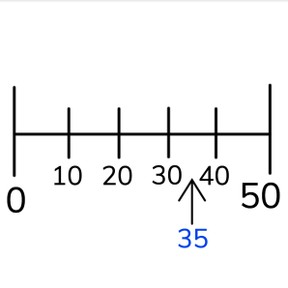Approximate placement of numbers to 50 on the number line

# Approximate placement of numbers to 50 on the number line

Approximate placement of numbers to 50 on the number line

No account needed.8,000 schools use Gynzy92,000 teachers use Gynzy1,600,000 students use Gynzy

## General

Students learn to approximately place numbers to 50 on the number line. They do so on a blank number line only showing 0 and 50.

K.CC.A.1
K.CC.A.2

## Relevance

Discuss with students that it is important to be able to approximately place numbers on the number line. If you know where a number approximately should be, it helps to determine if a number is bigger or smaller.

## Introduction

Explain that a number line is a line which shows numbers in their correct position. Ask students to drag tens numbers to 50 to the number line. Skip count in 10s forwards and backwards to 50 as a class. Ask students which neighboring tens numbers the given number sits between. Students then play a game using skip counting in 10s. In the first number line, all students start counting at 10. In the next number line, they start at 30. You can choose to hide the tens numbers with the cover. Count together as a class as they jump.

## Development

Show students a number line with intermediate lines and one that is blank and ask students what the difference between the two is. Discuss that on the number line on the left they can exactly see where a number belongs, and that you can't be as precise on the number line on the right. Explain the term "approximate", and say that it means you don't know the thing exactly. Tell students that when you have an empty number line, you first must find the middle. The middle of 0 and 50 is at 25. Place a number on the number line by first finding the middle, and then deciding if the number is bigger than, or smaller than the middle. Then determine the neighboring tens numbers and approximate placement. The first number that you practice as a class is smaller than 25. With the next numbers, have students first find the middle, then deciding if it is bigger or smaller, finding the neighboring tens numbers, and finally the approximate placement. Students can choose from multiple answers.

To check students understanding of approximate placement of numbers to 50 on the number line, you can ask the following questions:
- What are the numbers that our number line starts at and ends at?
- Skip count in 10s forwards and backwards to 50.
- What are steps you can take when approximating placement on the number line? (finding the middle, drawing imaginary tens lines)
- If you have an empty number line to 50, which number is the first number you should place when trying to approximately place a number? (25, find the middle)

## Guided practice

Students first practice with placing numbers on different empty number lines. First in jumps of 10, with the middle marked for them. Next they are given an empty number line with multiple jumps of 10 and a middle marker. Finally they place more difficult numbers.

## Closure

Discuss that knowing approximate placement of numbers to 50 on the number line is important, because you not only know where numbers belong, but also prepare to know when numbers are bigger and smaller than a given number. Check that students take the correct steps when approximating placement of a number on the blank number line: Finding the middle, looking if it is above or below the middle, deciding the neighboring tens numbers, and then placing the number. Play a game with the students of "Guess my number" with a number between 0 and 50. Students ask questions based on the order of placing a number. They use these steps to figure out which number you have in your head. Write their questions and steps on the board to help support the process.

## Teaching tips

Students who have difficulty with approximate placement of numbers can be supported by making their own number line to 50 and using this as they work on their exercises. You can also practice finding neighboring tens numbers with these students. They might also be supported by practicing the number line and recognizing the tens numbers.

## Instruction materials

Number cards from 0 to 50

### The online teaching platform for interactive whiteboards and displays in schools

• Save time building lessons

• Manage the classroom more efficiently

• Increase student engagement